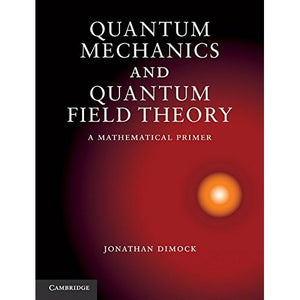># Quantum Mechanics and Quantum Field Theory: A Mathematical Primer

• £34.39
• Save £20

Jonathan Dimock
Cambridge University Press, 2011-02-03
EAN 9781107005099, ISBN10: 1107005094

Hardcover, 238 pages, 25.3 x 19.4 x 1.3 cm
Language: English

Explaining the concepts of quantum mechanics and quantum field theory in a precise mathematical language, this textbook is an ideal introduction for graduate students in mathematics, helping to prepare them for further studies in quantum physics. The textbook covers topics that are central to quantum physics: non-relativistic quantum mechanics, quantum statistical mechanics, relativistic quantum mechanics and quantum field theory. There is also background material on analysis, classical mechanics, relativity and probability. Each topic is explored through a statement of basic principles followed by simple examples. Around 100 problems throughout the textbook help readers develop their understanding.